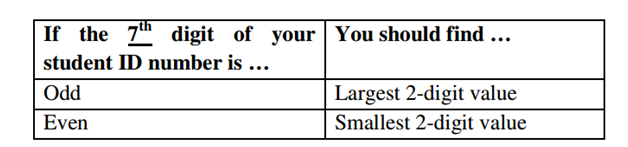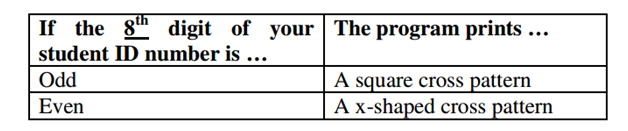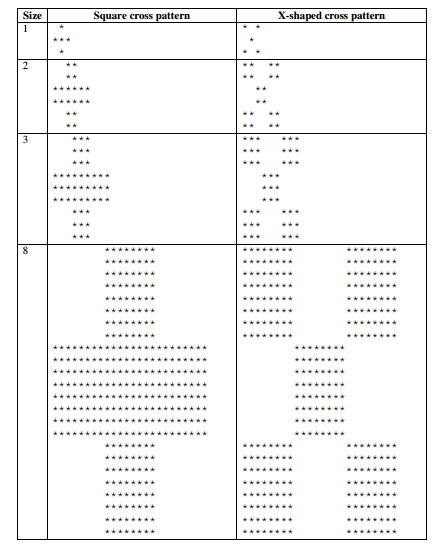# Creating C++ programs

Students of programming will always be required to create C++ programs. We provide help with C++ assignment so that any time a student gets stuck on an assignment from this programming language, they can get quality assistance.

## 3 small C++ programs

#### Introduction

You are given a C++ program template file calledA1Template.cpp. You are required to insert C++ codes into the template file according to thegiven instructions. The final program should be able to satisfy all the requirements in thisspecification.Complete the function Q1, so that when the user enters 1 in the Program Selection Menu, the
program performs the calculation with the following requirements:
1R1 Use the messages below to prompt for the user’s input of the coordinates of two points.
The user inputs the x- and y-coordinate of a point in the same line separated by a space:
Input (x1, y1): 3.2 1.5
Input (x2, y2): 17.5 14.2
1R2 According to the equation you use in this question, the program calculates either distance
or angle, and displays the result using the following output messages (e.g. input
coordinates as shown in 1R1):
The distance is 19.125 units
or
1R3 Following 1R2 above, the calculation result should be displayed in 3 decimal places.
1R4 After showing the calculation result, question 1 completes and the program returns back
1R5 Make sure that your program implements the correct equation (based on your student ID
number), and the calculation is correct.
1R6 You can assume that the users always enter numbers as the input for all the coordinates.

#### Question 2: Number Extraction

In this question, a positive integer value is considered. By checking every 2 consecutive digits
form the value, you are going to find the largest or smallest 2-digit value according to the table
below:Complete the function Q2, so that when the user enters 2 in the Program Selection Menu, the
program finds the required value with the following requirements:
2R1 Use the messages below to prompt for the user’s input of any positive integer value with
2 to 9 digits:
Input a positive integer with 2 to 9 digits: 462056843
2R2 Following 2R1 above, if the user inputs an invalid value, the following error message
should be displayed, and the program should allow user to input again until a correct
Error: Out-of-range. Input again: 4
Error: Out-of-range. Input again: 1462056843
Error: Out-of-range. Input again: -46205
Error: Out-of-range. Input again: 462056843
2R3 According to the value you find in this question, the program displays the 2-digit result
using the following output messages (e.g. input value as shown in 2R1):
The largest 2-digit value is 84
or
The smallest 2-digit value is 05
2R4 After showing the result, question 2 completes and the program returns back to the
2R5 Make sure that your program finds the correct 2-digit value (largest or smallest) based on
2R6 Appropriate use of loop is required in your codes.
2R7 You can assume that the users always enter integers when being asked for input, and the
first digit of the input number is a non-zero value.

#### Question 3: Cross

In this question, you are going to prints blocks of stars according to the size input by the user.
The pattern to be generated depends on whether the 8th digit of your student ID number is odd or
even, according to the table below:Complete the function Q3, so that when the user enters 3 in the Program Selection Menu, the
program prints the required pattern with the following requirements:
3R1 Use the messages below to prompt for the user’s input of size within the range 1 to 10,
inclusively:
Input size (1 to 10): 4
3R2 Following 2R1 above, if the user inputs an invalid size, the following error message
should be displayed, and the program should allow user to input again until a correct size
Error: Out-of-range. Input again: 13
Error: Out-of-range. Input again: 0
Error: Out-of-range. Input again: -5
Error: Out-of-range. Input again: 4
3R3 Note the meaning of size in the pattern. Make sure that the number of rows and the
number of stars in each row are printed correctly.
3R4 After printing the pattern, question 3 completes and the program returns back to the
3R5 Make sure that your program prints the correct pattern (square cross or x-shaped cross)
based on your student ID number.
3R6 Appropriate use of nested loop is required in your codes.
3R7 You can assume that the users always enter integers when being asked for input.

Example printouts of the cross pattern#### Solution

#include

#include

#include

using namespace std;

voidshowInfo()

{

cout<<“Name : XXX YYY ZZZ”<

cout<<“Student ID: 15251196A”<

cout<<“Class : 101A”<

cout<

}

void Q1()

{

cout<<“Input (x1, y1): “;

int x1,y1,x2,y2;

cin>>x1>>y1;

cout<

cout<<“Input (x2, y2): “;

cin>>x2>>y2;

cout<

double d=sqrt(((y2-y1)*(y2-y1))+((x2-x1)*(x2-x1)));

cout.setf(ios::fixed,ios::floatfield);

cout.precision(3);

cout<< “The distance is “<< d <<” units”<

cout<

}

void Q2()

{

long long x, x1, x2, x3, x4, x5, x6, x7, x8;

cout<<“Input a positive integer with 2 to 9 digits: “;

cin>>x;

while(x<10 || x>999999999)

{

cout<<“Error: Out-of-range. Input again: “;

cin>>x;

}

intarr;

arr = x/10000000;

arr = x/1000000 %100;

arr = x/100000 %100;

arr = x/10000 %100;

arr = x/1000 %100;

arr = x/100 %100;

arr = x/10 %100;

arr = x %100;

intans=0;

for(int i=0;i<9;i++)

{

ans=max(ans,arr[i]);

}

cout<<“The largest 2-digit value is “<

}

void Q3()

{

cout<<“Input size (1 to 10): “;

intn,m;

cin>>n;

while(n<1||n>10)

{

cout<

cout<<“Error: Out-of-range. Input again: “;

cin>>m;

n=m;

}

for(int i=0;i

{

for(int i=0;i<(3*n);i++)

{

if(i>=n&&i<(2*n))

{

cout<<” “;

}

else

cout<<“* “;

}

cout<

}

for(int i=0;i

{

for(int i=0;i<(2*n);i++)

{

if(i

cout<<” “;

else

cout<<“* “;

}

cout<

}

for(int i=0;i

{

for(int i=0;i<(3*n);i++)

{

if(i>=n&&i<(2*n))

{

cout<<” “;

}

else

cout<<“* “;

}

cout<

}

}

// GIVEN CODES, DO NOT MODIFY

// BEGIN

int main()

{

intprog_choice;

showInfo();

do {

cout<

cout<< “Assignment One – Program Selection Menu” <

cout<< “—————————————” <

cout<< “(1) Program One” <

cout<< “(2) Program Two” <

cout<< “(3) Program Three” <

cout<< “(4) Exit” <

cout<< “Enter the choice: “;

cin>>prog_choice;

switch (prog_choice){

case 1: Q1(); break;

case 2: Q2(); break;

case 3: Q3(); break;

case 4: break;

default:

cout<< “Please enter choice 1 – 4 only.” <

break;

}

} while (prog_choice != 4);

cout<< “Program terminates. Good bye!” <

return 0;

}

// END